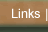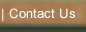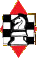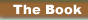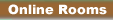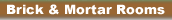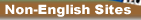The Maths Behind Poker

# The Maths Behind PokerTrying to decide what to play at the casino can be a tricky process - there's a great selection of card games from Betfair to choose from, including blackjack, baccarat and poker, but how well do you know the odds of those games? Do you know how to predict what's likely to be coming out next?

Never fear, we have this handy visual guide that walks you through working out the probabilities and odds involved in card games (we mostly use poker as the simplest example, but the rules apply to any game that uses a 52 deck of cards).

### Step 1: Understanding Odds and Probabilities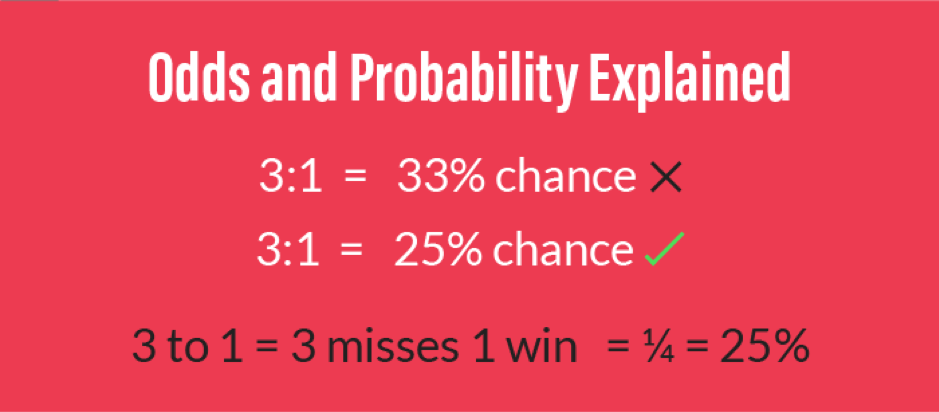Before we get started, we need to decide how we are going to represent probabilities. There are a couple of good options, but we've decided to use percentages as it's the easiest way to show probability without any confusion.

The problem comes from a common misunderstanding of how to read something like 3:1. It's surprisingly common that this is read as 33% instead of 25% with people believing 3 to 1 is synonymous with 1 in 3. It makes sense when you break it down with 3:1 being 3 losses for every 1 win so it's 1 in 4 or 25% instead.

In order to avoid confusion, we're sticking to percentage representation for easier understanding and so you can be confident in what the odds are whenever you see them.

### Step 2: Understanding Card Probabilities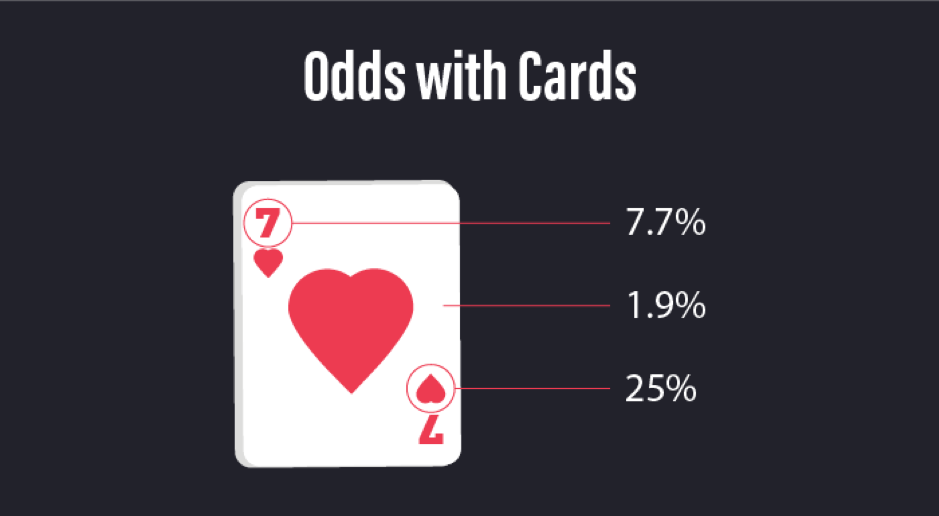With 52 cards in the deck, you work out the probability of being dealt something by dividing the total number in the deck by 52.

It's 1/52 for a card of a set value and suit, equalling about 1.9%, which is the probability of being dealt that particular card.

If you're after a certain value (a 7 for instance) then there are 4 in the deck but with 13 possible values, so it's 4/52 or 1/13 which is 7.7%.

For a card of a given suit, it's 13/52 or 1/4 so it's a 25% chance.

The important thing to remember is that, as cards are dealt, the odds will alter and you need to subtract from your calculation any known cards that have been played: this is most easily explained by using a single deck game such as poker.

### Step 3: Understanding Poker OutsIn poker, the outs are the cards you need to be dealt in order for your hand to reach maximum strength. For instance, if you hold two spades and there are two more on the turn then you only need one more to come out in order to have a flush. Even if you already have three of a kind or a pair, a flush is stronger and more likely to win. So, the out in this case is any spade.

You might assume the odds are 25%, but with 6 cards already out, you need to subtract that from the percentage. You know 6 cards have been dealt, so there are 46 remaining and 4 of them are the suit you need so there are 9 suited cards left.

9/46 gives you the percentage chance you have of receiving the card you need, which is around 19%.

When it comes to calculating this, you only count the cards you've seen, meaning the other players' hands aren't taken into consideration (because you can't know what they are).

### Step 4: Quick Calculations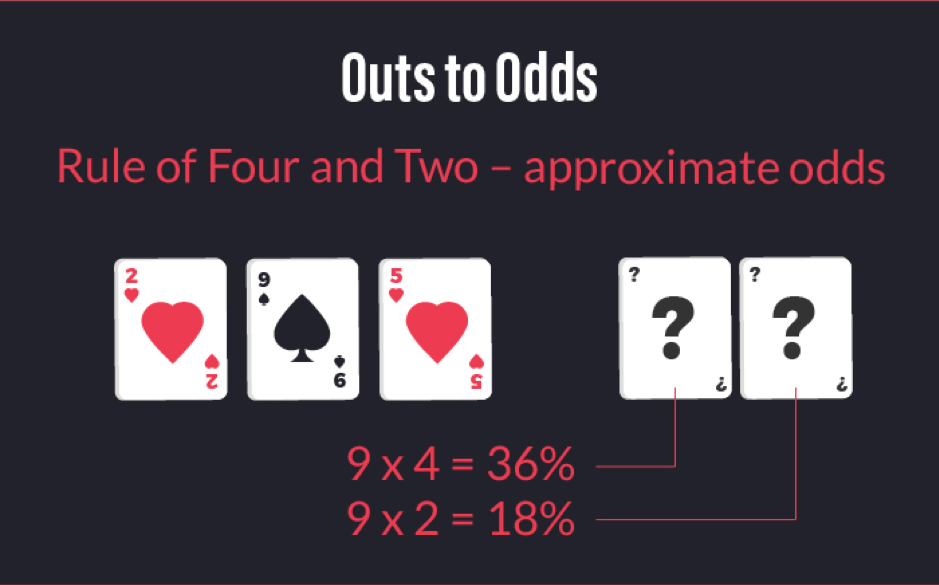The rule of four and two is a useful method to approximate odds while playing. You won't get the exact probability, or you're very unlikely to, but it should be close enough for you to make a choice. This is how to put it into practice - when you've only seen the flop, you multiply the out by 4 and if you've just seen the turn you multiply by 2.

Looking at the results you get, you might be curious as to why our previous estimate of 19% has been replaced by 36% for the first draw and 18% for the second. As you have two chances of the card drawn being the one you need, the probability is doubled for the first draw but with just one card left to come out, it then reverts to 18% for the next.

While it isn't exact, it gives you a good approximation.

### Step 5: Other Games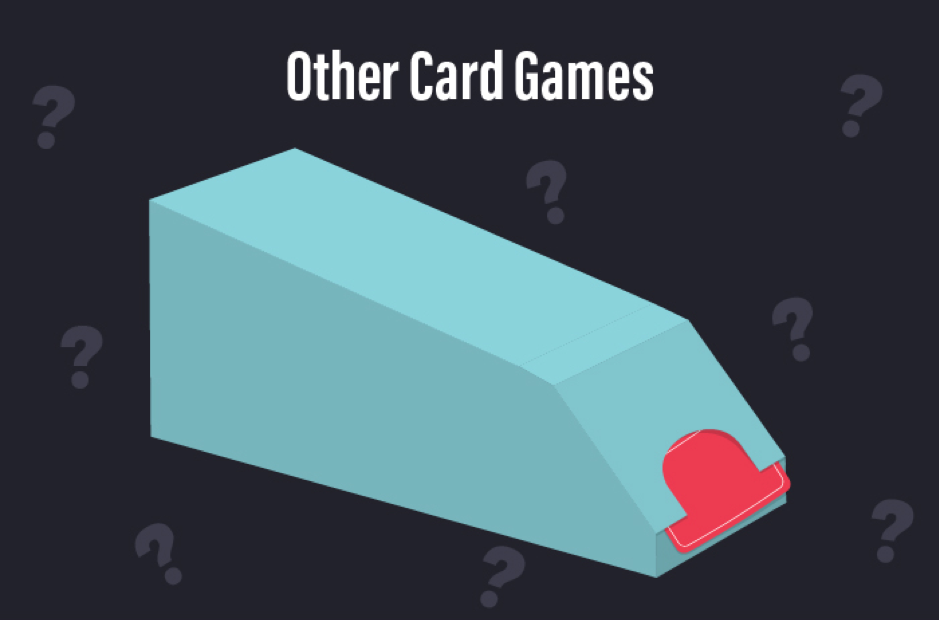That's all well and good for a single deck game like poker, but what about other popular casino games? The ones that need multiple decks. You can't calculate an out with as much reliability because it's nearly impossible to keep track of what's gone before (the multiple deck idea is to limit card counting which is a similar technique), but the general X/52 calculation should hold. You may have more luck when it comes to mobile casino games though - rather than multiple decks, these tend to use random number generator software and can 'shuffle' a deck in between each deal so trying to calculate when your next card is coming is simple.

Do you think you're ready to put this into practice? Why not play a few hands and see how you do!